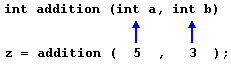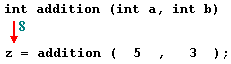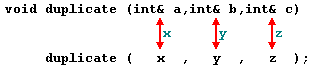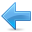# Functions

Functions allow to structure programs in segments of code to perform individual tasks.

In C++, a function is a group of statements that is given a name, and which can be called from some point of the program. The most common syntax to define a function is:

``` type name ( parameter1, parameter2, ...) { statements } ```
Where:
- `type` is the type of the value returned by the function.
- `name` is the identifier by which the function can be called.
- `parameters` (as many as needed): Each parameter consists of a type followed by an identifier, with each parameter being separated from the next by a comma. Each parameter looks very much like a regular variable declaration (for example: `int x`), and in fact acts within the function as a regular variable which is local to the function. The purpose of parameters is to allow passing arguments to the function from the location where it is called from.
- `statements` is the function's body. It is a block of statements surrounded by braces { } that specify what the function actually does.

Let's have a look at an example:

 ``1234567891011121314151617`` ``````// function example #include using namespace std; int addition (int a, int b) { int r; r=a+b; return r; } int main () { int z; z = addition (5,3); cout << "The result is " << z; }`````` `The result is 8`

This program is divided in two functions: `addition` and `main`. Remember that no matter the order in which they are defined, a C++ program always starts by calling `main`. In fact, `main` is the only function called automatically, and the code in any other function is only executed if its function is called from `main` (directly or indirectly).

In the example above, `main` begins by declaring the variable `z` of type `int`, and right after that, it performs the first function call: it calls `addition`. The call to a function follows a structure very similar to its declaration. In the example above, the call to `addition` can be compared to its definition just a few lines earlier:The parameters in the function declaration have a clear correspondence to the arguments passed in the function call. The call passes two values, `5` and `3`, to the function; these correspond to the parameters `a` and `b`, declared for function `addition`.

At the point at which the function is called from within main, the control is passed to function `addition`: here, execution of `main` is stopped, and will only resume once the `addition` function ends. At the moment of the function call, the value of both arguments (`5` and `3`) are copied to the local variables `int a` and `int b` within the function.

Then, inside `addition`, another local variable is declared (`int r`), and by means of the expression `r=a+b`, the result of `a` plus `b` is assigned to `r`; which, for this case, where `a` is 5 and `b` is 3, means that 8 is assigned to `r`.

The final statement within the function:

 `` `` ``return r;``

Ends function `addition`, and returns the control back to the point where the function was called; in this case: to function `main`. At this precise moment, the program resumes its course on `main` returning exactly at the same point at which it was interrupted by the call to `addition`. But additionally, because `addition` has a return type, the call is evaluated as having a value, and this value is the value specified in the return statement that ended `addition`: in this particular case, the value of the local variable `r`, which at the moment of the `return` statement had a value of 8.Therefore, the call to `addition` is an expression with the value returned by the function, and in this case, that value, 8, is assigned to `z`. It is as if the entire function call (`addition(5,3)`) was replaced by the value it returns (i.e., 8).

Then main simply prints this value by calling:

 `` `` ``cout << "The result is " << z;``

A function can actually be called multiple times within a program, and its argument is naturally not limited just to literals:

 ``123456789101112131415161718192021`` ``````// function example #include using namespace std; int subtraction (int a, int b) { int r; r=a-b; return r; } int main () { int x=5, y=3, z; z = subtraction (7,2); cout << "The first result is " << z << '\n'; cout << "The second result is " << subtraction (7,2) << '\n'; cout << "The third result is " << subtraction (x,y) << '\n'; z= 4 + subtraction (x,y); cout << "The fourth result is " << z << '\n'; }`````` ```The first result is 5 The second result is 5 The third result is 2 The fourth result is 6```

Similar to the `addition` function in the previous example, this example defines a `subtract` function, that simply returns the difference between its two parameters. This time, `main` calls this function several times, demonstrating more possible ways in which a function can be called.

Let's examine each of these calls, bearing in mind that each function call is itself an expression that is evaluated as the value it returns. Again, you can think of it as if the function call was itself replaced by the returned value:

 ``12`` ``````z = subtraction (7,2); cout << "The first result is " << z;``````

If we replace the function call by the value it returns (i.e., 5), we would have:

 ``12`` ``````z = 5; cout << "The first result is " << z;``````

With the same procedure, we could interpret:
 `` `` ``cout << "The second result is " << subtraction (7,2);``

as:
 `` `` ``cout << "The second result is " << 5;``

since 5 is the value returned by `subtraction (7,2)`.

In the case of:

 `` `` ``cout << "The third result is " << subtraction (x,y);``

The arguments passed to subtraction are variables instead of literals. That is also valid, and works fine. The function is called with the values `x` and `y` have at the moment of the call: 5 and 3 respectively, returning 2 as result.

The fourth call is again similar:

 `` `` ``z = 4 + subtraction (x,y);``

The only addition being that now the function call is also an operand of an addition operation. Again, the result is the same as if the function call was replaced by its result: 6. Note, that thanks to the commutative property of additions, the above can also be written as:

 `` `` ``z = subtraction (x,y) + 4;``

With exactly the same result. Note also that the semicolon does not necessarily go after the function call, but, as always, at the end of the whole statement. Again, the logic behind may be easily seen again by replacing the function calls by their returned value:

 ``12`` ``````z = 4 + 2; // same as z = 4 + subtraction (x,y); z = 2 + 4; // same as z = subtraction (x,y) + 4; ``````

### Functions with no type. The use of void

The syntax shown above for functions:

``` type name ( argument1, argument2 ...) { statements } ```
Requires the declaration to begin with a type. This is the type of the value returned by the function. But what if the function does not need to return a value? In this case, the type to be used is `void`, which is a special type to represent the absence of value. For example, a function that simply prints a message may not need to return any value:

 ``12345678910111213`` ``````// void function example #include using namespace std; void printmessage () { cout << "I'm a function!"; } int main () { printmessage (); }`````` `I'm a function!`

`void` can also be used in the function's parameter list to explicitly specify that the function takes no actual parameters when called. For example, `printmessage` could have been declared as:

 ``1234`` ``````void printmessage (void) { cout << "I'm a function!"; }``````

In C++, an empty parameter list can be used instead of `void` with same meaning, but the use of `void` in the argument list was popularized by the C language, where this is a requirement.

Something that in no case is optional are the parentheses that follow the function name, neither in its declaration nor when calling it. And even when the function takes no parameters, at least an empty pair of parentheses shall always be appended to the function name. See how `printmessage` was called in an earlier example:

 `` `` ``printmessage ();``

The parentheses are what differentiate functions from other kinds of declarations or statements. The following would not call the function:

 `` `` ``printmessage;``

### The return value of main

You may have noticed that the return type of `main` is `int`, but most examples in this and earlier chapters did not actually return any value from `main`.

Well, there is a catch: If the execution of `main` ends normally without encountering a `return` statement the compiler assumes the function ends with an implicit return statement:

 `` `` ``return 0;``

Note that this only applies to function `main` for historical reasons. All other functions with a return type shall end with a proper `return` statement that includes a return value, even if this is never used.

When `main` returns zero (either implicitly or explicitly), it is interpreted by the environment as that the program ended successfully. Other values may be returned by `main`, and some environments give access to that value to the caller in some way, although this behavior is not required nor necessarily portable between platforms. The values for `main` that are guaranteed to be interpreted in the same way on all platforms are:

valuedescription
`0`The program was successful
`EXIT_SUCCESS`The program was successful (same as above).
This value is defined in header `<cstdlib>`.
`EXIT_FAILURE`The program failed.
This value is defined in header `<cstdlib>`.

Because the implicit `return 0;` statement for `main` is a tricky exception, some authors consider it good practice to explicitly write the statement.

### Arguments passed by value and by reference

In the functions seen earlier, arguments have always been passed by value. This means that, when calling a function, what is passed to the function are the values of these arguments on the moment of the call, which are copied into the variables represented by the function parameters. For example, take:

 ``12`` ``````int x=5, y=3, z; z = addition ( x, y );``````

In this case, function addition is passed 5 and 3, which are copies of the values of `x` and `y`, respectively. These values (5 and 3) are used to initialize the variables set as parameters in the function's definition, but any modification of these variables within the function has no effect on the values of the variables x and y outside it, because x and y were themselves not passed to the function on the call, but only copies of their values at that moment.In certain cases, though, it may be useful to access an external variable from within a function. To do that, arguments can be passed by reference, instead of by value. For example, the function `duplicate` in this code duplicates the value of its three arguments, causing the variables used as arguments to actually be modified by the call:

 ``123456789101112131415161718`` ``````// passing parameters by reference #include using namespace std; void duplicate (int& a, int& b, int& c) { a*=2; b*=2; c*=2; } int main () { int x=1, y=3, z=7; duplicate (x, y, z); cout << "x=" << x << ", y=" << y << ", z=" << z; return 0; }`````` `x=2, y=6, z=14`

To gain access to its arguments, the function declares its parameters as references. In C++, references are indicated with an ampersand (`&`) following the parameter type, as in the parameters taken by `duplicate` in the example above.

When a variable is passed by reference, what is passed is no longer a copy, but the variable itself, the variable identified by the function parameter, becomes somehow associated with the argument passed to the function, and any modification on their corresponding local variables within the function are reflected in the variables passed as arguments in the call.In fact, `a`, `b`, and `c` become aliases of the arguments passed on the function call (`x`, `y`, and `z`) and any change on `a` within the function is actually modifying variable `x` outside the function. Any change on `b` modifies `y`, and any change on `c` modifies `z`. That is why when, in the example, function `duplicate` modifies the values of variables `a`, `b`, and `c`, the values of `x`, `y`, and `z` are affected.

If instead of defining duplicate as:

 `` `` ``void duplicate (int& a, int& b, int& c) ``

Was it to be defined without the ampersand signs as:

 `` `` ``void duplicate (int a, int b, int c)``

The variables would not be passed by reference, but by value, creating instead copies of their values. In this case, the output of the program would have been the values of `x`, `y`, and `z` without being modified (i.e., 1, 3, and 7).

### Efficiency considerations and const references

Calling a function with parameters taken by value causes copies of the values to be made. This is a relatively inexpensive operation for fundamental types such as `int`, but if the parameter is of a large compound type, it may result on certain overhead. For example, consider the following function:

 ``1234`` ``````string concatenate (string a, string b) { return a+b; }``````

This function takes two strings as parameters (by value), and returns the result of concatenating them. By passing the arguments by value, the function forces `a` and `b` to be copies of the arguments passed to the function when it is called. And if these are long strings, it may mean copying large quantities of data just for the function call.

But this copy can be avoided altogether if both parameters are made references:

 ``1234`` ``````string concatenate (string& a, string& b) { return a+b; }``````

Arguments by reference do not require a copy. The function operates directly on (aliases of) the strings passed as arguments, and, at most, it might mean the transfer of certain pointers to the function. In this regard, the version of `concatenate` taking references is more efficient than the version taking values, since it does not need to copy expensive-to-copy strings.

On the flip side, functions with reference parameters are generally perceived as functions that modify the arguments passed, because that is why reference parameters are actually for.

The solution is for the function to guarantee that its reference parameters are not going to be modified by this function. This can be done by qualifying the parameters as constant:

 ``1234`` ``````string concatenate (const string& a, const string& b) { return a+b; }``````

By qualifying them as `const`, the function is forbidden to modify the values of neither `a` nor `b`, but can actually access their values as references (aliases of the arguments), without having to make actual copies of the strings.

Therefore, `const` references provide functionality similar to passing arguments by value, but with an increased efficiency for parameters of large types. That is why they are extremely popular in C++ for arguments of compound types. Note though, that for most fundamental types, there is no noticeable difference in efficiency, and in some cases, const references may even be less efficient!

### Inline functions

Calling a function generally causes a certain overhead (stacking arguments, jumps, etc...), and thus for very short functions, it may be more efficient to simply insert the code of the function where it is called, instead of performing the process of formally calling a function.

Preceding a function declaration with the `inline` specifier informs the compiler that inline expansion is preferred over the usual function call mechanism for a specific function. This does not change at all the behavior of a function, but is merely used to suggest the compiler that the code generated by the function body shall be inserted at each point the function is called, instead of being invoked with a regular function call.

For example, the concatenate function above may be declared inline as:

 ``1234`` ``````inline string concatenate (const string& a, const string& b) { return a+b; }``````

This informs the compiler that when `concatenate` is called, the program prefers the function to be expanded inline, instead of performing a regular call. `inline` is only specified in the function declaration, not when it is called.

Note that most compilers already optimize code to generate inline functions when they see an opportunity to improve efficiency, even if not explicitly marked with the `inline` specifier. Therefore, this specifier merely indicates the compiler that inline is preferred for this function, although the compiler is free to not inline it, and optimize otherwise. In C++, optimization is a task delegated to the compiler, which is free to generate any code for as long as the resulting behavior is the one specified by the code.

### Default values in parameters

In C++, functions can also have optional parameters, for which no arguments are required in the call, in such a way that, for example, a function with three parameters may be called with only two. For this, the function shall include a default value for its last parameter, which is used by the function when called with fewer arguments. For example:

 ``1234567891011121314151617`` ``````// default values in functions #include using namespace std; int divide (int a, int b=2) { int r; r=a/b; return (r); } int main () { cout << divide (12) << '\n'; cout << divide (20,4) << '\n'; return 0; }`````` ```6 5```

In this example, there are two calls to function `divide`. In the first one:

 `` `` ``divide (12)``

The call only passes one argument to the function, even though the function has two parameters. In this case, the function assumes the second parameter to be 2 (notice the function definition, which declares its second parameter as `int b=2`). Therefore, the result is 6.

In the second call:

 `` `` ``divide (20,4)``

The call passes two arguments to the function. Therefore, the default value for `b` (`int b=2`) is ignored, and `b` takes the value passed as argument, that is 4, yielding a result of 5.

### Declaring functions

In C++, identifiers can only be used in expressions once they have been declared. For example, some variable `x` cannot be used before being declared with a statement, such as:

 `` `` ``int x;``

The same applies to functions. Functions cannot be called before they are declared. That is why, in all the previous examples of functions, the functions were always defined before the `main` function, which is the function from where the other functions were called. If `main` were defined before the other functions, this would break the rule that functions shall be declared before being used, and thus would not compile.

The prototype of a function can be declared without actually defining the function completely, giving just enough details to allow the types involved in a function call to be known. Naturally, the function shall be defined somewhere else, like later in the code. But at least, once declared like this, it can already be called.

The declaration shall include all types involved (the return type and the type of its arguments), using the same syntax as used in the definition of the function, but replacing the body of the function (the block of statements) with an ending semicolon.

The parameter list does not need to include the parameter names, but only their types. Parameter names can nevertheless be specified, but they are optional, and do not need to necessarily match those in the function definition. For example, a function called `protofunction` with two int parameters can be declared with either of these statements:

 ``12`` ``````int protofunction (int first, int second); int protofunction (int, int);``````

Anyway, including a name for each parameter always improves legibility of the declaration.

 ``1234567891011121314151617181920212223242526272829`` ``````// declaring functions prototypes #include using namespace std; void odd (int x); void even (int x); int main() { int i; do { cout << "Please, enter number (0 to exit): "; cin >> i; odd (i); } while (i!=0); return 0; } void odd (int x) { if ((x%2)!=0) cout << "It is odd.\n"; else even (x); } void even (int x) { if ((x%2)==0) cout << "It is even.\n"; else odd (x); }`````` ```Please, enter number (0 to exit): 9 It is odd. Please, enter number (0 to exit): 6 It is even. Please, enter number (0 to exit): 1030 It is even. Please, enter number (0 to exit): 0 It is even.```

This example is indeed not an example of efficiency. You can probably write yourself a version of this program with half the lines of code. Anyway, this example illustrates how functions can be declared before its definition:

The following lines:

 ``12`` ``````void odd (int a); void even (int a); ``````

Declare the prototype of the functions. They already contain all what is necessary to call them, their name, the types of their argument, and their return type (`void` in this case). With these prototype declarations in place, they can be called before they are entirely defined, allowing for example, to place the function from where they are called (`main`) before the actual definition of these functions.

But declaring functions before being defined is not only useful to reorganize the order of functions within the code. In some cases, such as in this particular case, at least one of the declarations is required, because `odd` and `even` are mutually called; there is a call to `even` in `odd` and a call to `odd` in `even`. And, therefore, there is no way to structure the code so that `odd` is defined before `even`, and `even` before `odd`.

### Recursivity

Recursivity is the property that functions have to be called by themselves. It is useful for some tasks, such as sorting elements, or calculating the factorial of numbers. For example, in order to obtain the factorial of a number (`n!`) the mathematical formula would be:

`n! = n * (n-1) * (n-2) * (n-3) ... * 1`
More concretely, `5!` (factorial of 5) would be:

`5! = 5 * 4 * 3 * 2 * 1 = 120`
And a recursive function to calculate this in C++ could be:

 ``123456789101112131415161718`` ``````// factorial calculator #include using namespace std; long factorial (long a) { if (a > 1) return (a * factorial (a-1)); else return 1; } int main () { long number = 9; cout << number << "! = " << factorial (number); return 0; }`````` `9! = 362880`

Notice how in function factorial we included a call to itself, but only if the argument passed was greater than 1, since, otherwise, the function would perform an infinite recursive loop, in which once it arrived to 0, it would continue multiplying by all the negative numbers (probably provoking a stack overflow at some point during runtime).Previous:Statements and flow controlIndexNext:Overloads and templates